Next: Building blocks of the Up: Ensemble learning Previous: Ensemble learning   Contents

## Information theoretic approach

In their original paper , Hinton and van Camp approached ensemble learning from an information theoretic point of view by using the Minimum Description Length (MDL) Principle . They developed a new coding method for noisy parameter values which led to the cost function of Equation (3.11). This allows interpreting the cost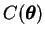in Equation (3.11) as a description length for the data using the chosen model.

The MDL principle asserts that the best model for given data is the one that attains the shortest description of the data. The description length can be evaluated in bits and it represents the length of the message needed to transmit the data. The idea is that one builds a model for the data and then sends the description of that model and the residual of the data that could not be modelled. Thus the total description length is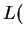data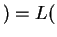model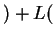error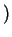.

The code length is related to probability because according to the coding theorem, an event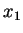having probability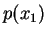can be coded using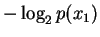bits, assuming both the sender and the receiver know the distribution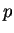.

In their article Hinton and van Camp developed a method for encoding the parameters of the model in such a way, that the expected code length is the one given by Equation (3.11). Derivation of this result can be found in the original paper by Hinton and van Camp  or in the doctoral thesis  by Harri Valpola.Next: Building blocks of the Up: Ensemble learning Previous: Ensemble learning   Contents
Antti Honkela 2001-05-30